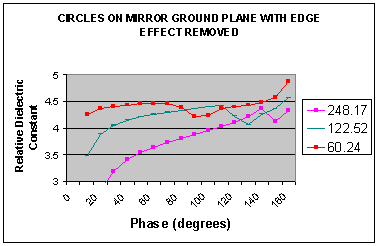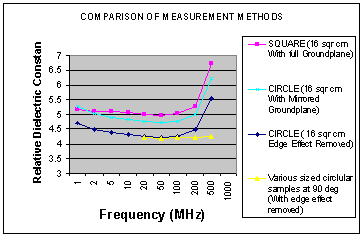# Measuring Relative Dielectric Constant of PCB's Using Parallel Plate Method and a Vector Network Analyser page 5

Maximum Size

The maximum size of a sample can be derived from a study of the three largest circles as shown in Figure 10.

Figure 10.There are three peaks that occur at 140, 110 and 70 degrees. If these are examined in relation to frequency, there is a common result. They all occur just below the frequency that the proximity effect starts. This frequency is calculated the same as skin effect.

From Transmission Lines and Networks by Walter C. Johnson, McGraw-Hill 1963 p58.

For a copper conductor

δ = 6.64 / f   Formula 5.

Where   δ = nominal depth of penetration in cm

f = frequency in Hertz

The copper on the sample board is 1/2 oz per square foot or 0.00175 cm thick. The proximity effect can be calculated as follows.

f = (6.64 / 0.00175)2 = 14.4 MHz

Table 1.

 Area (cm2) Phase at Peak (degrees) Next Highest Phase (degrees) Frequency at Peak (MHz) Next Highest Frequency (MHz) 248.17 s140 150 13.63 19.35 122.52 110 120 13.915 17.536 60.24 70 80 13.489 16.361

It can be seen in Table1, that the peak is just below the point at which the proximity effect starts. It can be concluded from this that the maximum size of the sample is dependant on the thickness of the copper plane.

We have not been able to obtain samples locally with a different copper thickness to prove that the frequency changes with copper thickness.

Minimum Size

This is more difficult to assess and it is probable that the errors present with a small sample are due to such things as the ratio of the drilled hole and countersink in relation to the overall size. The amount of solder applied and the general difficulties of measuring at more than 1 GHz. It is most likely that any sample under 1 cm radius would be unsuitable regardless of the materials used.

Conclusion

It can be seen from this study that the sample size, shape and mirror ground are all important. As is the connection method, port extension and sample connection point (central). The inaccuracies that can occur are considerable and comparisons are made in Figure 11.

Figure 11.The minimum error for a 16cm2 square with full ground plane is 18.5% at 50MHz, and this is probably typical of actual samples used by the unaware. If a microstrip transmission line of a required 50Ω is calculated from the resultant εr an actual characteristic impedance of 46.32Ω will be achieved.

Another point to note is that the Relative Dielectric Constant is just as it name implies, it is a constant and does not change very much at all over frequency. The combination of various circle sizes measured at 900 in Figure 11 shows a straight line (within measurement accuracy limits). As we have now observed, a curve over a frequency band is indicative of measurement error not a change in εr. It is not important to measure at a set frequency, although εr is typically quoted by PCB manufacturers at 1 MHz. The proximity effect has not been established properly at such a low frequency and the figure should be regarded as suspect. Most equations available to calculate track dimensions do not use frequency and the εr is assumed to be constant over frequency. It is very important that the measurement is made at 90° (regardless of frequency).

It is also important to get the right sample size and to assist with this, we have produced a PCB Sample Size Calculator for relative dielectric constant measurements. If in doubt start with a circle of 2.5 cm radius, this should cover most materials available.

1  2  3  4  5  >  Page

Technical Article IndexW J Highton 24/10/2004